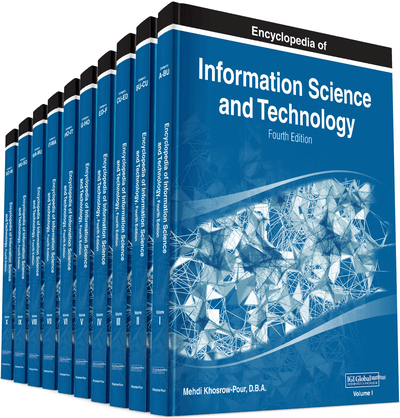# Moving Object Detection and Tracking Based on the Contour Extraction and Centroid Representation

Naveenkumar M (National Institute of Technology Trichy, India), Sriharsha K. V. (National Institute of Technology Trichy, India) and Vadivel A (National Institute of Technology Trichy, India)
DOI: 10.4018/978-1-5225-2255-3.ch019

## Abstract

This chapter presents a novel approach for moving object detection and tracking based on the Contour Extraction and Centroid Representation (CECR). Firstly, two consecutive frames are read from the video and they are converted into gray scale. Next, the absolute difference is calculated between them and the result frame is converted into binary by applying gray threshold technique. The binary frame is segmented using contour extraction algorithm. The centroid representation is used for motion tracking. In the second stage of experiment, initially object is detected by using CECR and motion of each track is estimated by kalman filter. Experimental results show that the proposed method can robustly detect and track the moving object.
Chapter Preview
Top

## Object Tracking Using Contour Extraction And Centroid Representation

A primitive experiment is conducted on single object moving with a constant speed and no occlusions. In this perspective a series of videos captured using Nikon COOLPIX 12.0 mega pixels are used for motion analysis. For moving object identification, frame differencing technique is chosen. In this technique, absolute difference between two successive frames i and i+1 is calculated. For each of the differenced image that is obtained in each of the successive iteration, a grey threshold is calculated and is applied. As a result the differenced images are transformed into binary images. To smooth the binary image, normalized box filter is applied and then again grey threshold is calculated. As a result, the perfect binary image is produced. For each of the moving object that is identified in the preprocessed image, a centroid is computed. This centroid represents the moving object in each of the differenced image. Finally a trajectory is drawn by connecting the centroids for all the differenced images. The results obtained after testing the algorithm in a video is presented for discussion. The pseudo code for object detection and tracking using Contour Extraction and Centroid Representation (CECR) is shown below.

## Key Terms in this Chapter

State Vector: Set of parameters describing a system, known as states, which the Kalman filter estimates.

Correction Step: Uses the current value of the estimate to refine the result given by the predictor step.

Object Representation: In a tracking scenario, an object can be defined as anything that is of interest for further analysis.

Prediction Step: Calculates the next estimate of the state based only on past measurements of the output.

Thresolding: Separates the regions of the image corresponding to objects in which we are interested, from the regions of the image that correspond to background.

Prior State: State during time span (ti-1 to t).

Object Detection: Identifying an object over a sequence of frames in a video.

Object Tracking: It is defined as the problem of estimating the trajectory of an object in an image plane as it moves around a scene.

Measurement Vector: It is a set of simultaneous measurements of properties of the system which are functions of the state vector.

Covariance Measure: Covariance measures the degree to which two variables change or vary together (i.e. co-vary).

Posterior State: State during time span (ti+1 to t).

Grayscale Images (or Gray Level): Are simply whose colors are the shades of gray. Each pixel in a gray scale image is represented only with single intensity value (stored as 8 bit integer value).

System Noise (Q): Determines the variation in the true values of the states.

Measurement Noise(R): Associated with the measurement vector, describes the statistics of the noise on the measurements.

Image Filtering: It is the process of transforming pixel intensity values to reveal certain image characteristics like image enhancement, smoothing technique and template matching.

Kalman Gain Matrix (K): Determine the weighting of the measurement information in updating the state estimates.

Observation Matrix: It is a measure of how dependent the measurements are upon the state of the system.

Measurement Residue: Gives difference in measurement between the true state vector and the estimated state vector.

Kalman Filter: A recursive predictive filter used for estimating Kalman filter is used to estimate the state of a linear system where the state is assumed to be distributed by a Gaussian.

Error Covariance Matrix (?P): Defines the expectation of the square of the deviation of the state vector estimate from the true value of the state vector.

State Transformation Matrix (A): It is an approximation of the change that the state undergoes over the specified time interval.

## Complete Chapter List

Search this Book:
Reset